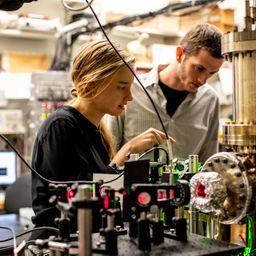## 2.5 \times 10^{20} \text { photons }

Quantum Physics

Atomic Physics

### Discussion

You must be signed in to discuss.
##### Top Physics 103 Educators##### Christina K.

Rutgers, The State University of New Jersey##### Andy C.

University of Michigan - Ann Arbor##### Aspen F.

University of Sheffield

### Video Transcript

Hey, this is an interesting question. I am not sure if I see the broader picture of God's gonna roll with it. So we have a two kilogram object, falls five meters to the ground. Um, so, um is two kilograms and start reminds me it's one object on the distance. It falls is five meters from the Sync. Fix was at 5.0. And to find out okay, and then we know that if we convert this energy to a certain wavelengths we want about how many protons air produced. Okay, let's kind of start with this. What can you get from here? I mean, we can figure out how much kinetic energy is gained because, um, if you consider the system of the object and then the earth, then, um, energy, there's no external forces acting on it. And so energy is conserved. So you can say the change in kinetic energy is equal to the change in potential energy. And then the change in potential energy is equal to M g. D. So that's how you get the changing kinetic energy, and then that's gonna be a certain number, and then we want to set it equal to the total energy from photons of that wavelength. So we just had Lambda was equal to 500 nanometers were all destroyed it down 5.0 times 10 to the minus seven. And so we're sending people to this total energy of protons of that type that's going to be number four times protons time. Use the energy for proton. That's the energy for Proton. So and is gonna be nostalgia, Brickley manipulating mg de times lambda provided by each time. See? And now I'm gonna go ahead and combat into a calculator and kind of long, small possibility while I do that. Okay, so I got 2.5 times 10 of the 20 protons, all right?University of Washington

#### Topics

Quantum Physics

Atomic Physics

##### Top Physics 103 Educators##### Christina K.

Rutgers, The State University of New Jersey##### Andy C.

University of Michigan - Ann Arbor##### Aspen F.

University of Sheffield